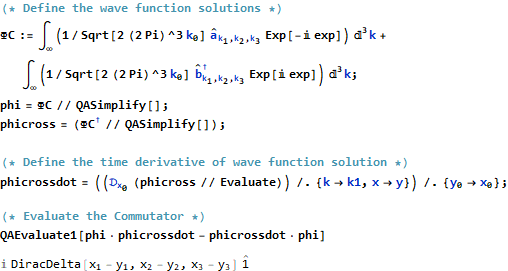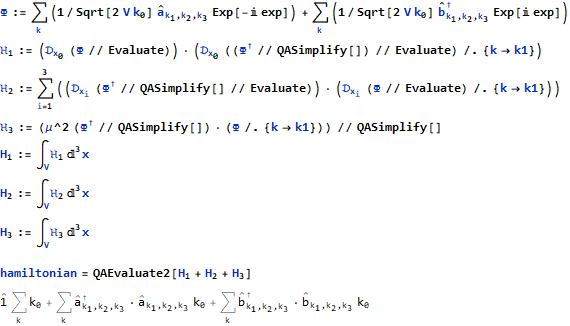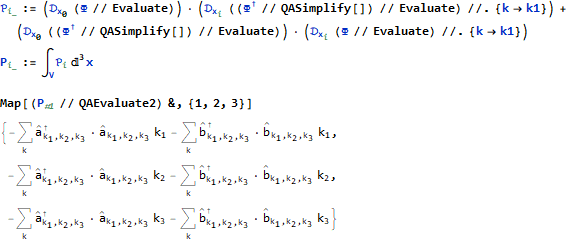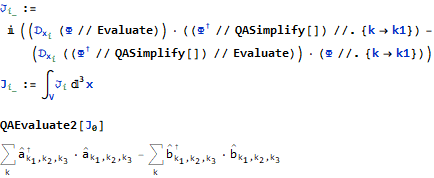## Sample Applications

The sample applications are given in the form of notebooks. For the time being there are the following:

• A notebook for the Klein - Gordon - equation
• A notebook for the Dirac - equation

In addition to local rules, they contain a series of calculations, e.g. Hamilton - operator, 3 - momentum and evaluation of charge.

### Klein - Gordon - Equation

In all cases, the free fields that solve the field equations are specified.

1. The commutator of the fields, when applying the commutation relations for the coefficients of the fields, leads to the commutation relations of the fields themselves.2. The Hamiltonian as an expression dependent on the fields leads to an expression in the coefficients of the fields (creation and annihilation operators).3. The same applies to the expression of the 3-momentum.4. The same applies to the expression for the charge, which results from a continuity equation.